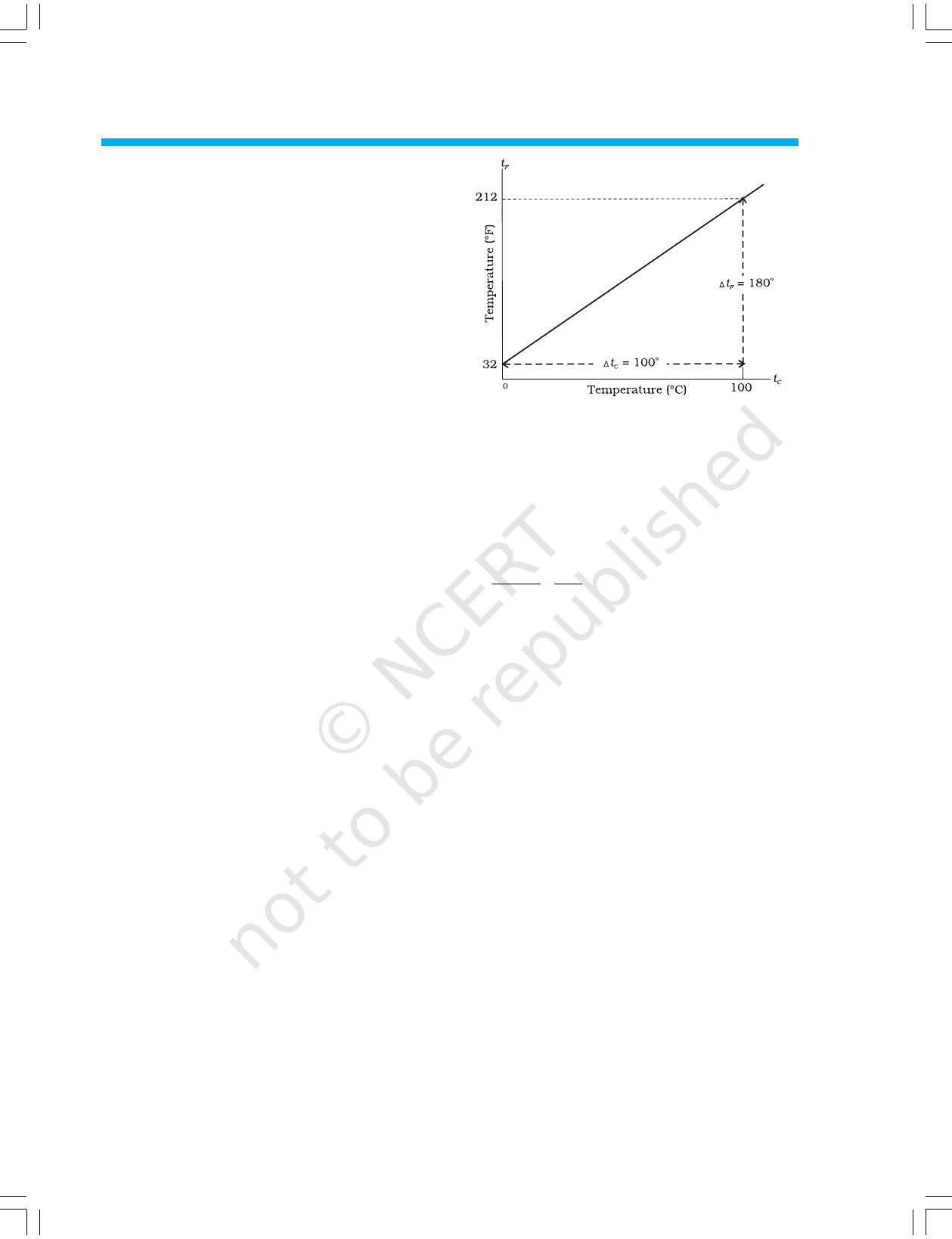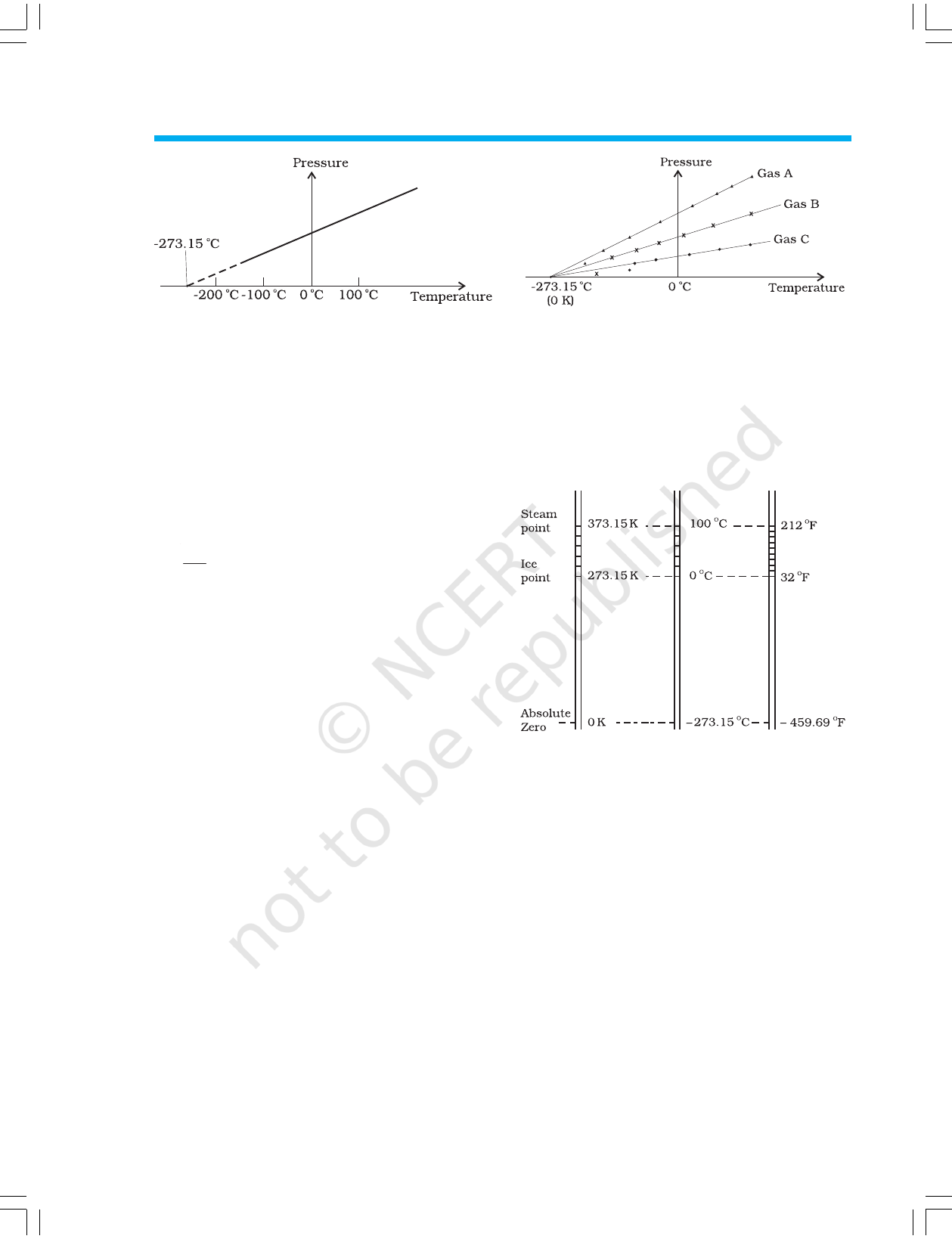CHAPTER ELEVEN
THERMAL PROPERTIES OF MATTER
11.1 INTRODUCTION
We all have common sense notions of heat and temperature.
Temperature is a measure of ‘hotness’ of a body. A kettle
with boiling water is hotter than a box containing ice. In
physics, we need to define the notion of heat, temperature,
etc., more carefully. In this chapter, you will learn what heat
is and how it is measured, and study the various proceses by
which heat flows from one body to another. Along the way,
you will find out why blacksmiths heat the iron ring before
fitting on the rim of a wooden wheel of a horse cart and why
the wind at the beach often reverses direction after the sun
goes down. You will also learn what happens when water boils
or freezes, and its temperature does not change during these
processes even though a great deal of heat is flowing into or
out of it.
11.2 TEMPERATURE AND HEAT
We can begin studying thermal properties of matter with
definitions of temperature and heat. Temperature is a relative
measure, or indication of hotness or coldness. A hot utensil
is said to have a high temperature, and ice cube to have a
low temperature. An object that has a higher temperature
than another object is said to be hotter. Note that hot and
cold are relative terms, like tall and short. We can perceive
temperature by touch. However, this temperature sense is
somewhat unreliable and its range is too limited to be useful
for scientific purposes.
We know from experience that a glass of ice-cold water left
on a table on a hot summer day eventually warms up whereas
a cup of hot tea on the same table cools down. It means that
when the temperature of body, ice-cold water or hot tea in
this case, and its surrounding medium are different, heat
transfer takes place between the system and the surrounding
medium, until the body and the surrounding medium are at
the same temperature. We also know that in the case of glass
tumbler of ice-cold water, heat flows from the environment to
11.1 Introduction
11.2 Temperature and heat
11.3 Measurement of
temperature
11.4 Ideal-gas equation and
absolute temperature
11.5 Thermal expansion
11.6 Specific heat capacity
11.7 Calorimetry
11.8 Change of state
11.9 Heat transfer
11.10 Newton’s law of cooling
Summary
Points to ponder
Exercises
2020-21the glass tumbler, whereas in the case of hot
tea, it flows from the cup of hot tea to the
environment. So, we can say that heat is the
form of energy transferred between two (or
more) systems or a system and its
surroundings by virtue of temperature
difference. The SI unit of heat energy
transferred is expressed in joule (J) while SI unit
of temperature is Kelvin (K), and degree Celsius
(
o
C) is a commonly used unit of temperature.
When an object is heated, many changes may
take place. Its temperature may rise, it may
expand or change state. We will study the effect
of heat on different bodies in later sections.
11.3 MEASUREMENT OF TEMPERATURE
A measure of temperature is obtained using a
thermometer. Many physical properties of
materials change sufficiently with temperature.
Some such properties are used as the basis for
constructing thermometers. The commonly used
property is variation of the volume of a liquid
with temperature. For example, in common
liquid–in–glass thermometers, mercury, alcohol
etc., are used whose volume varies linearly with
temperature over a wide range.
Thermometers are calibrated so that a
numerical value may be assigned to a given
temperature in an appropriate scale. For the
definition of any standard scale, two fixed
reference points are needed. Since all
substances change dimensions with
temperature, an absolute reference for
expansion is not available. However, the
necessary fixed points may be correlated to the
physical phenomena that always occur at the
same temperature. The ice point and the steam
point of water are two convenient fixed points
and are known as the freezing and boiling
points, respectively. These two points are the
temperatures at which pure water freezes and
boils under standard pressure. The two familiar
temperature scales are the Fahrenheit
temperature scale and the Celsius temperature
scale. The ice and steam point have values
32 °F and 212 °F, respectively, on the Fahrenheit
scale and 0 °C and 100 °C on the Celsius scale.
On the Fahrenheit scale, there are 180 equal
intervals between two reference points, and on
the Celsius scale, there are 100.
Fig. 11.1 A plot of Fahrenheit temperature (t
F
) versus
Celsius temperature (t
c
).
A relationship for converting between the two
scales may be obtained from a graph of
Fahrenheit temperature (t
F
) versus celsius
temperature (t
C
) in a straight line (Fig. 11.1),
whose equation is
t
t
F
C
32
180 100
=
(11.1)
11.4 IDEAL-GAS EQUATION AND
ABSOLUTE TEMPERATURE
Liquid-in-glass thermometers show different
readings for temperatures other than the fixed
points because of differing expansion properties.
A thermometer that uses a gas, however, gives
the same readings regardless of which gas is
used. Experiments show that all gases at low
densities exhibit same expansion behaviour. The
variables that describe the behaviour of a given
quantity (mass) of gas are pressure, volume, and
temperature (P, V, and T)(where T = t + 273.15;
t is the temperature in °C). When temperature
is held constant, the pressure and volume of a
quantity of gas are related as PV = constant.
This relationship is known as Boyle’s law, after
Robert Boyle (1627–1691), the English Chemist
who discovered it. When the pressure is held
constant, the volume of a quantity of the gas is
related to the temperature as V/T = constant.
This relationship is known as Charles’ law,
after French scientist Jacques Charles (1747–
1823). Low-density gases obey these
laws, which may be combined into a single
THERMAL PROPERTIES OF MATTER 279
2020-21280 PHYSICS
relationship. Notice that since PV = constant
and V/T = constant for a given quantity of gas,
then PV/T should also be a constant. This
relationship is known as ideal gas law. It can be
written in a more general form that applies not
just to a given quantity of a single gas but to any
quantity of any low-density gas and is known as
ideal-gas equation:
PV
R
T
µ
=
or PV =
µ
RT (11.2)
where,
µ
is the number of moles in the sample
of gas and R is called universal gas constant:
R = 8.31 J mol
–1
K
–1
In Eq. 11.2, we have learnt that the pressure
and volume are directly proportional to
temperature : PV T. This relationship allows a
gas to be used to measure temperature in a
constant volume gas thermometer. Holding the
volume of a gas constant, it gives P T. Thus,
with a constant-volume gas thermometer,
temperature is read in terms of pressure. A plot
of pressure versus temperature gives a straight
line in this case, as shown in Fig. 11.2.
However, measurements on real gases deviate
from the values predicted by the ideal gas law
at low temperature. But the relationship is linear
over a large temperature range, and it looks as
though the pressure might reach zero with
decreasing temperature if the gas continued to
be a gas. The absolute minimum temperature
for an ideal gas, therefore, inferred by
extrapolating the straight line to the axis, as in
Fig. 11.3. This temperature is found to be
– 273.15 °C and is designated as absolute zero.
Absolute zero is the foundation of the Kelvin
temperature scale or absolute scale temperature
named after the British scientist Lord Kelvin. On
this scale, – 273.15 °C is taken as the zero point,
that is 0 K (Fig. 11.4).
The size of unit in Kelvin and Celsius
temperature scales is the same. So, temperature
on these scales are related by
T = t
C
+ 273.15 (11.3)
11.5 THERMAL EXPANSION
You may have observed that sometimes sealed
bottles with metallic lids are so tightly screwed
that one has to put the lid in hot water for some
time to open it. This would allow the metallic lid
to expand, thereby loosening it to unscrew
easily. In case of liquids, you may have observed
that mercury in a thermometer rises, when the
thermometer is put in slightly warm water. If
we take out the thermometer from the warm
Fig. 11.2 Pressure versus temperature of a low
density gas kept at constant volume.
Fig. 11.3 A plot of pressure versus temperature and
extrapolation of lines for low density gases
indicates the same absolute zero
temperature.
Fig. 11.4 Comparision of the Kelvin, Celsius and
Fahrenheit temperature scales.
2020-21